Examples

Chapter 6 Class 12 Application of Derivatives
Serial order wise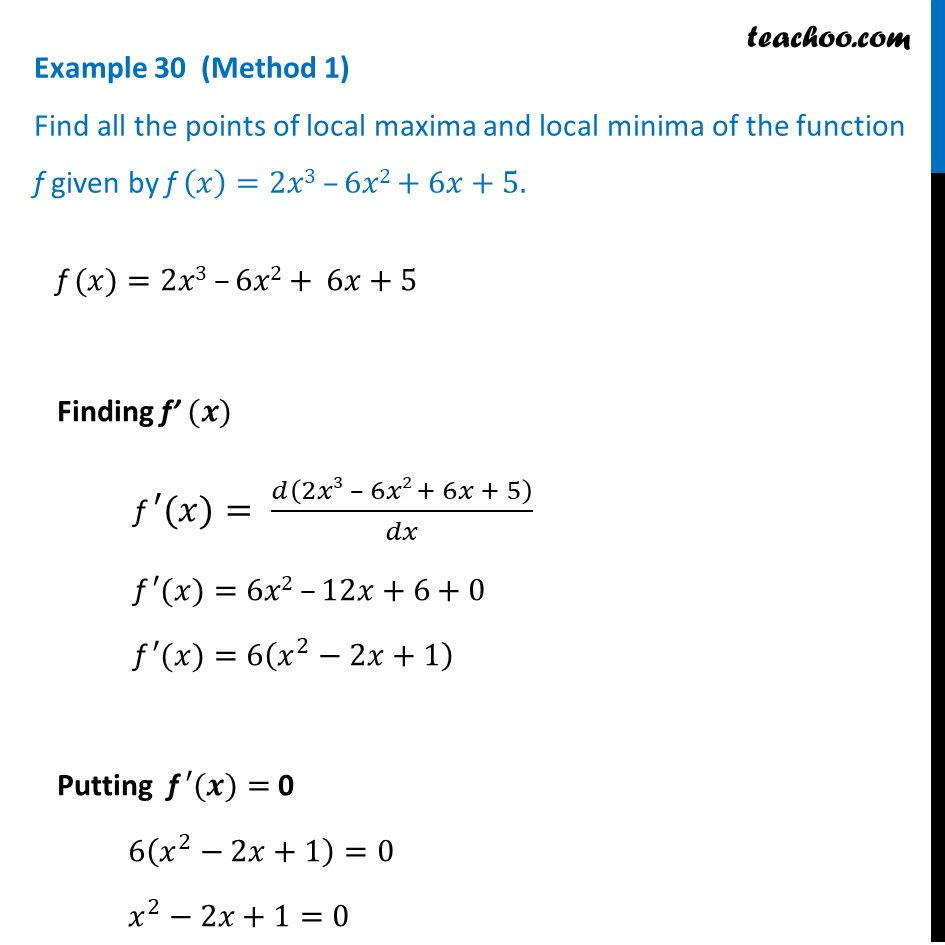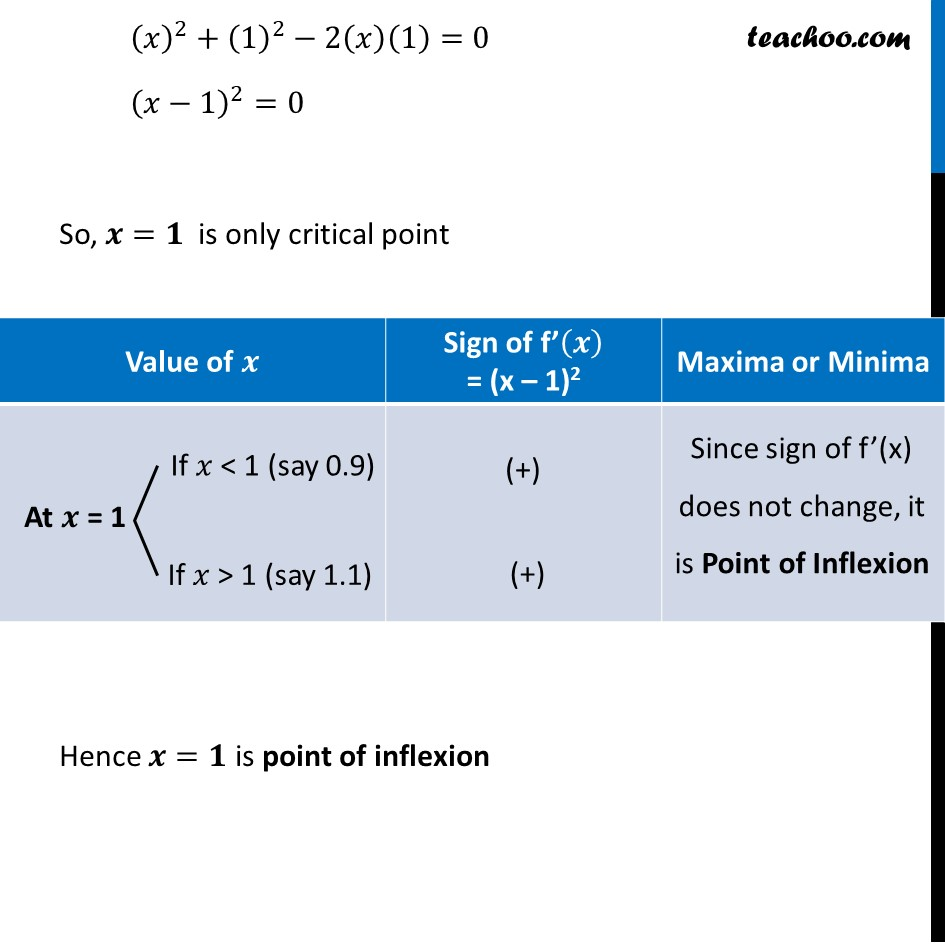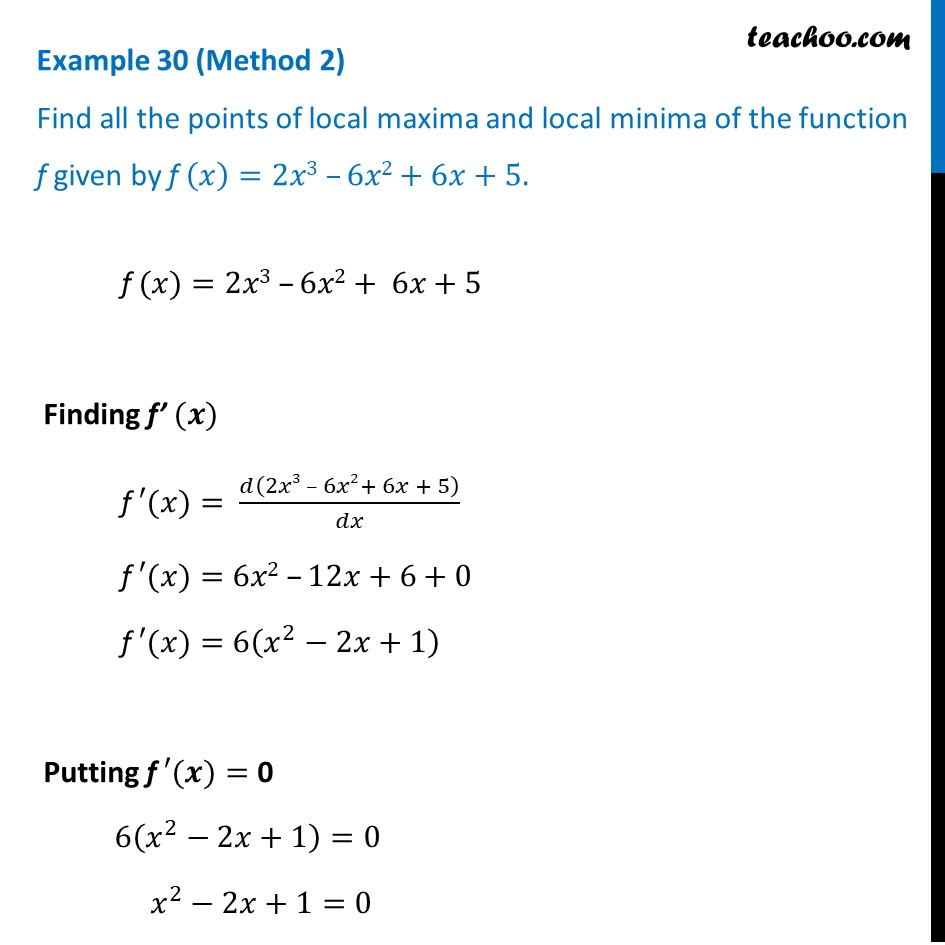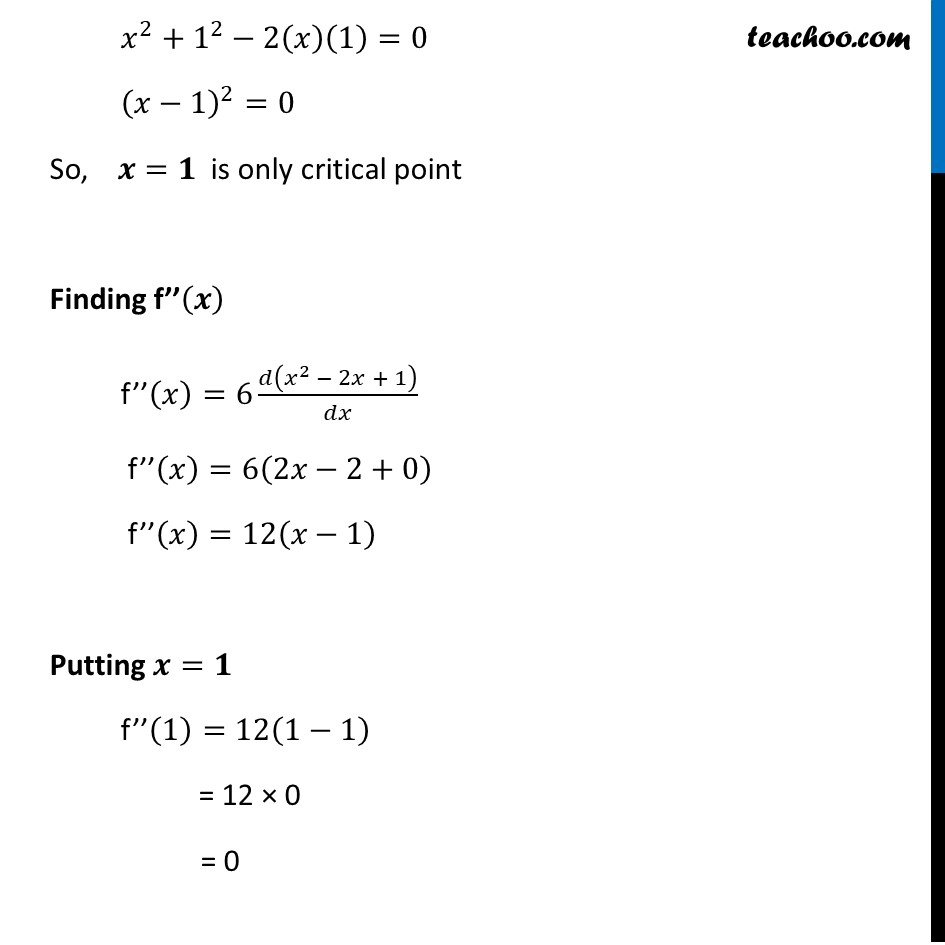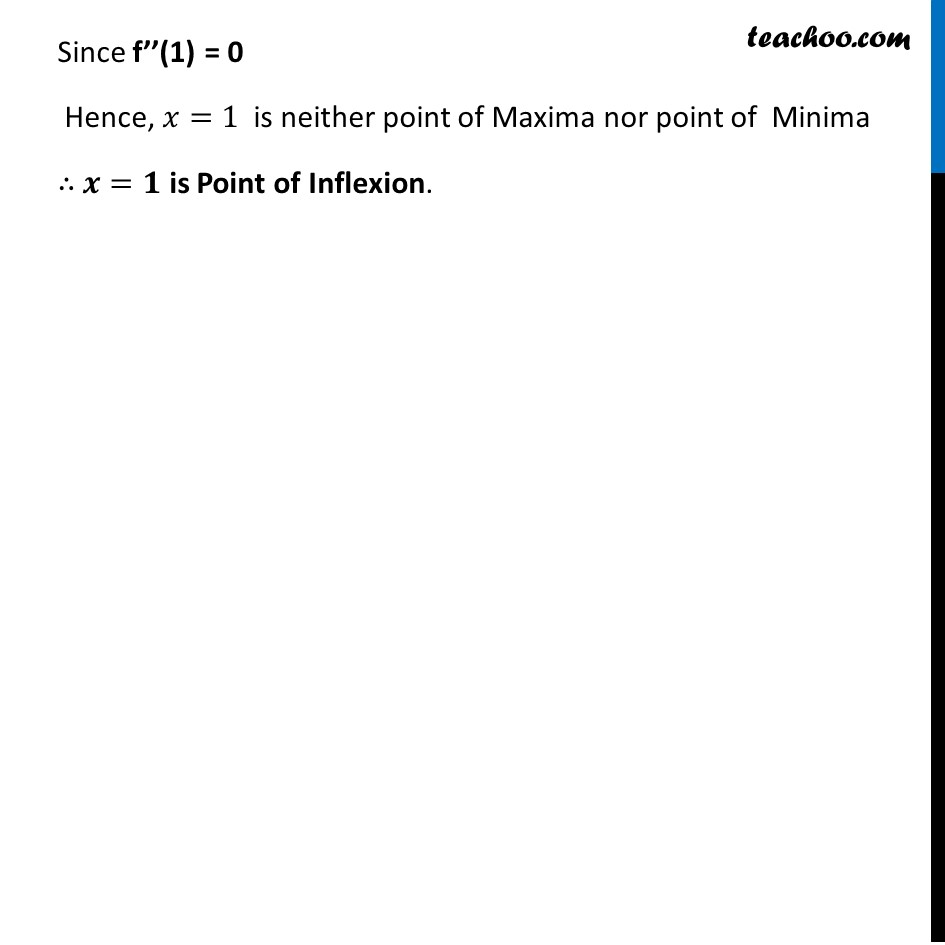Get live Maths 1-on-1 Classs - Class 6 to 12

### Transcript

Example 30 (Method 1) Find all the points of local maxima and local minima of the function f given by f (𝑥)=2𝑥3 –6𝑥2+6𝑥+5.f (𝑥)=2𝑥3 –6𝑥2+ 6𝑥+5 Finding f’ (𝒙) f ′(𝑥)= 𝑑(2𝑥3 – 6𝑥2 + 6𝑥 + 5)/𝑑𝑥 f ′(𝑥)=6𝑥2 –12𝑥+6+0 f ′(𝑥)=6(𝑥^2−2𝑥+1) Putting f ′(𝒙)= 0 6(𝑥^2−2𝑥+1)=0 𝑥^2−2𝑥+1=0 (𝑥)^2+(1)^2−2(𝑥)(1)=0 (𝑥−1)^2=0 So, 𝒙=𝟏 is only critical point Hence 𝒙=𝟏 is point of inflexion Example 30 (Method 2) Find all the points of local maxima and local minima of the function f given by f (𝑥)=2𝑥3 –6𝑥2+6𝑥+5. f (𝑥)=2𝑥3 –6𝑥2+ 6𝑥+5 Finding f’ (𝒙) f ′(𝑥)= 𝑑(2𝑥3 – 6𝑥2+ 6𝑥 + 5)/𝑑𝑥 f ′(𝑥)=6𝑥2 –12𝑥+6+0 f ′(𝑥)=6(𝑥^2−2𝑥+1) Putting f ′(𝒙)= 0 6(𝑥^2−2𝑥+1)=0 𝑥^2−2𝑥+1=0 𝑥^2+1^2−2(𝑥)(1)=0 (𝑥−1)^2=0 So, 𝒙=𝟏 is only critical point Finding f’’(𝒙) f’’(𝑥)=6 𝑑(𝑥^2 − 2𝑥 + 1)/𝑑𝑥 f’’(𝑥)=6(2𝑥−2+0) f’’(𝑥)=12(𝑥−1) Putting 𝒙=𝟏 f’’(1)=12(1−1) = 12 × 0 = 0 Since f’’(1) = 0 Hence, 𝑥=1 is neither point of Maxima nor point of Minima ∴ 𝒙=𝟏 is Point of Inflexion.